# Logistic regression

## 11 Oct 2016

Damjan Vukcevic
University of Melbourne

## Linear regression## Linear regression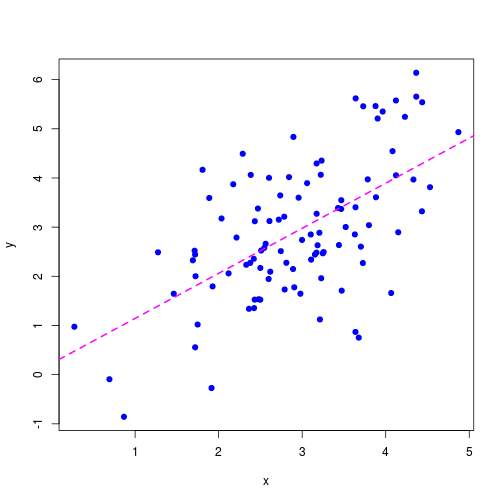## Binary outcome variable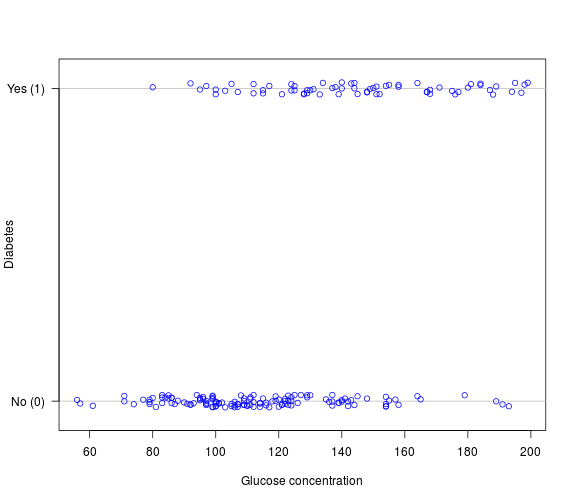## Binary outcome variable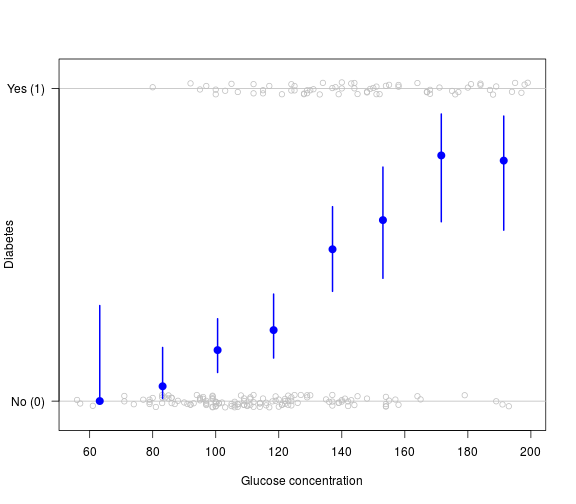## Binary outcome variable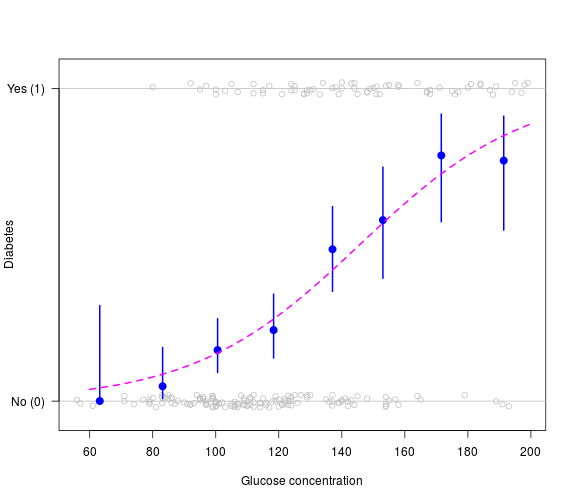## Binary outcome variable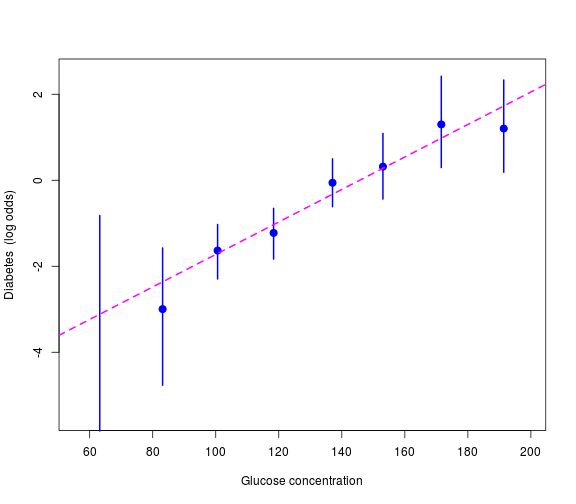## Logit transformation

Transform outcome probabilities onto the log odds scale: $\mathrm{logit}(p) = \log\left(\frac{p}{1-p}\right)$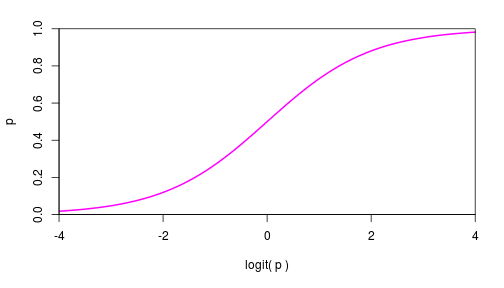Such a transformation is called a link function

## Logistic regression

$Y_i$ is binary outcome variable (taking values 0 or 1)
$X_i$ is a predictor variable

Logistic regression model: $p_i = \Pr(Y_i = 1 \mid X_i)$ $\mathrm{logit}(p_i) = \log\left(\frac{p_i}{1-p_i}\right) = \mu + \beta X_i$

$Y_i$ are independent, conditional on $X_i$

## Logistic regression

$Y_i$ is binary outcome variable (taking values 0 or 1)
$X_i$ is a predictor variable

Logistic regression model: $p_i = \Pr(Y_i = 1 \mid X_i)$ $\mathrm{logit}(p_i) = \log\left(\frac{p_i}{1-p_i}\right) = \mu + \beta X_i$

$Y_i$ are independent, conditional on $X_i$

Note:

• $\mathrm{logit()}$ ensures all predictions give valid probabilities,
by 'linking' the outcome scale ($p_i$) with the modelling scale ($\mu + \beta X_i$)

## Example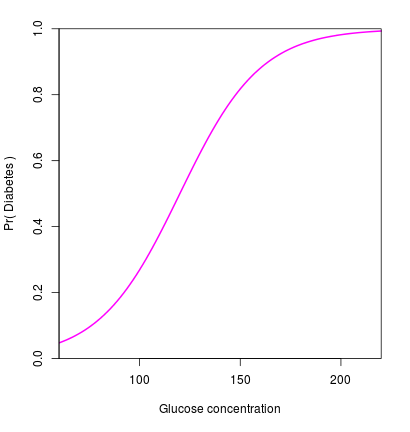Let $\mu = -6$ and $\beta = 0.05$, $\mathrm{logit}(p_i) = -6 + 0.05 \, X_i$

## ExampleLet $\mu = -6$ and $\beta = 0.05$, $\mathrm{logit}(p_i) = -6 + 0.05 \, X_i$

Re-arrange, $p_i = \frac{e^{-6 + 0.05 X_i}}{1 + e^{-6 + 0.05 X_i}}$

## Example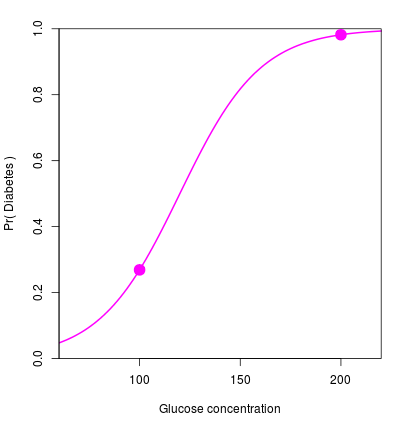Let $\mu = -6$ and $\beta = 0.05$, $\mathrm{logit}(p_i) = -6 + 0.05 \, X_i$

Re-arrange, $p_i = \frac{e^{-6 + 0.05 X_i}}{1 + e^{-6 + 0.05 X_i}}$

Plug in some values: $X_i = 100 \quad \mapsto \quad p_i = 0.27$ $X_i = 200 \quad \mapsto \quad p_i = 0.98$

## Parameter interpretation

Re-write the model: \begin{aligned} \mathrm{logit}(p_i) &= \mu + \beta X_i \\ \Rightarrow \quad \frac{p_i}{1 - p_i} &= e^{\mu + \beta X_i} \end{aligned}

## Parameter interpretation

Re-write the model: \begin{aligned} \mathrm{logit}(p_i) &= \mu + \beta X_i \\ \Rightarrow \quad \frac{p_i}{1 - p_i} &= e^{\mu + \beta X_i} \end{aligned}

Consider binary $X_i \in \{0, 1\}$ $X_i = 0 \quad \Rightarrow \quad \frac{p_i}{1 - p_i} = e^{\mu} \phantom{e^{\beta}}$ $X_i = 1 \quad \Rightarrow \quad \frac{p_i}{1 - p_i} = e^{\mu} e^{\beta}$

## Parameter interpretation

Re-write the model: \begin{aligned} \mathrm{logit}(p_i) &= \mu + \beta X_i \\ \Rightarrow \quad \frac{p_i}{1 - p_i} &= e^{\mu + \beta X_i} \end{aligned}

Consider binary $X_i \in \{0, 1\}$ $X_i = 0 \quad \Rightarrow \quad \frac{p_i}{1 - p_i} = e^{\mu} \phantom{e^{\beta}}$ $X_i = 1 \quad \Rightarrow \quad \frac{p_i}{1 - p_i} = e^{\mu} e^{\beta}$

$e^{\mu}$ is the baseline odds

$e^{\beta}$ is the odds ratio (OR), and $\beta$ is the log odds ratio

## Comparison to linear regression

Logistic regression: $\mathrm{logit}\left(\Pr\left(Y_i = 1\right)\right) = \mu + \beta X_i$

Linear regression: $Y_i = \mu + \beta X_i + \epsilon_i$ $\epsilon_i \sim \mathrm{N}(0, \sigma^2)$

Differences:

• No error term
• No variance parameter

## Likelihood function

The likelihood is the probability of the observed outcome variables: $L(\mu, \beta) = \prod_i \Pr(Y_i = y_i \mid X_i = x_i)$

## Likelihood function

The likelihood is the probability of the observed outcome variables: $L(\mu, \beta) = \prod_i \Pr(Y_i = y_i \mid X_i = x_i)$

In this case, Bernoulli random variables: $L(\mu, \beta) = \prod_i p_i^{y_i} (1 - p_i)^{1 - y_i}$

## Likelihood function

The likelihood is the probability of the observed outcome variables: $L(\mu, \beta) = \prod_i \Pr(Y_i = y_i \mid X_i = x_i)$

In this case, Bernoulli random variables: $L(\mu, \beta) = \prod_i p_i^{y_i} (1 - p_i)^{1 - y_i}$

The parameters are hidden inside the $p_i$ terms, $p_i = \frac{e^{\mu + \beta x_i}}{1 + e^{\mu + \beta x_i}}$

## Likelihood function

The likelihood is the probability of the observed outcome variables: $L(\mu, \beta) = \prod_i \Pr(Y_i = y_i \mid X_i = x_i)$

In this case, Bernoulli random variables: $L(\mu, \beta) = \prod_i p_i^{y_i} (1 - p_i)^{1 - y_i}$

The parameters are hidden inside the $p_i$ terms, $p_i = \frac{e^{\mu + \beta x_i}}{1 + e^{\mu + \beta x_i}}$

Maximise the likelihood using the iteratively reweighted least squares (IRLS) method. Estimation and testing then follow the same as for linear regression...

## Estimation

Parameter estimates from maximum likelihood
Standard error from the Fisher information matrix

Example: \begin{aligned} \hat\mu &= -5.5 &(\textrm{s.e. }\: 0.84) \\ \hat\beta &= 0.038 &(\textrm{s.e. }\: 0.0063) \end{aligned}

Easier to interpret as an odds ratio: $\textrm{OR} = e^{\hat\beta} = 1.04$

Can also calculate a confidence interval for the OR: $\textrm{95% CI} \approx e^{\hat\beta \pm 2 \mathrm{se}(\hat\beta)} = (1.03, 1.05)$

Standard likelihood theory: estimates are asymptotically unbiased, efficient and normally distributed

## Hypothesis testing

Usually interested in tests of effect parameters, for example: $\begin{cases} H_0\colon \: \beta = 0 \\ H_1\colon \: \beta \ne 0 \end{cases}$

Use a likelihood ratio test to carry this out:

• Fit both models
• Compare $-2 \log(\textrm{likelihood ratio})$ against a $\chi^2$ distribution
• Usually, just summarise the outcome by a p-value

## Hypothesis testing

Usually interested in tests of effect parameters, for example: $\begin{cases} H_0\colon \: \beta = 0 \\ H_1\colon \: \beta \ne 0 \end{cases}$

Use a likelihood ratio test to carry this out:

• Fit both models
• Compare $-2 \log(\textrm{likelihood ratio})$ against a $\chi^2$ distribution
• Usually, just summarise the outcome by a p-value

Example: $\textrm{p-value} = 2.5 \times 10^{-12}$

## Building up more complex models

Can add more predictor variables, the same as for linear regression:

• Continuous $x$
• Discrete $x$
• Non-linear transformations ($x^2$, $\sin(x)$, etc.)
• Interaction terms

For example: $\mathrm{logit}(p_i) = \mu + \beta_1 X_i + \beta_2 X_i^2 + \gamma_j \mathrm{I}(Z_i = j)$

## Case-control studies and logistic regression

Case-control studies: only ORs are estimable

Logistic regression: estimates ORs

A perfect match!

## Case-control studies and logistic regression

Case-control studies: only ORs are estimable

Logistic regression: estimates ORs

A perfect match!

Example: genome-wide association studies (GWAS)

## GWAS example, single SNP analysis

Consider a disease study with 1000 cases and 1000 controls

For individual $i$:

• $Y_i$ is the case-control status
• $G_i$ is the genotype of a given SNP

## GWAS example, single SNP analysis

Consider a disease study with 1000 cases and 1000 controls

For individual $i$:

• $Y_i$ is the case-control status
• $G_i$ is the genotype of a given SNP

Example data:

$G = 0$ $G = 1$ $G = 2$
$Y = 0$ (controls) 360 468 172
$Y = 1$ (cases) 301 486 213

## Models for single-SNP association

$\mathrm{logit}(p_i) = \mu + \beta G_i$ $G_i$ is numeric
Each copy of the SNP allele increases the odds of disease by $e^{\beta}$
This model underlies the vast majority of analyses

## Models for single-SNP association

$\mathrm{logit}(p_i) = \mu + \beta G_i$ $G_i$ is numeric
Each copy of the SNP allele increases the odds of disease by $e^{\beta}$
This model underlies the vast majority of analyses

### General model

$\mathrm{logit}(p_i) = \mu + \beta_1 \mathrm{I}(G_i = 1) + \beta_2 \mathrm{I}(G_i = 2)$ $G_i$ is categorical
The heterozygote OR is $e^{\beta_1}$ and the homozygote OR is $e^{\beta_2}$

## Inference with the additive model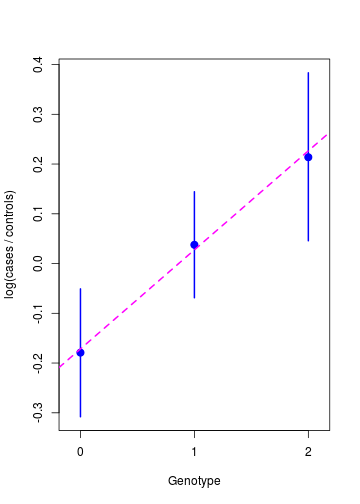Parameter estimates: \begin{aligned} \hat\mu &= -0.17 &(\textrm{s.e. }\: 0.071) \\ \hat\beta &= 0.20 &(\textrm{s.e. }\: 0.063) \end{aligned}

\begin{aligned} \textrm{OR} &= e^{\hat\beta} = 1.22 \\ \textrm{95% CI} &\approx (1.08, 1.38) \end{aligned}

Association test: $\begin{cases} H_0\colon \: \beta = 0 \\ H_1\colon \: \beta \ne 0 \end{cases}$

$\textrm{p-value} = 0.0017$

NOT 'genome-wide significant'

## Extensions

$\mathrm{logit}(p_i) = \mu + \beta X_i + \mathbf{\gamma Z}$

For example: sex, ethnicity, principal components,...

Inference and testing follows similarly

## Extensions

$\mathrm{logit}(p_i) = \mu + \beta X_i + \mathbf{\gamma Z}$